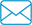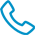800-871-5022

# Torque Conversion Chart Metric To English

TorqueConvMetricEnglish.pdf

 Newton Meters (N-m) Foot Pounds (ft. lbs.) Newton Meters (N-m) Foot Pounds (ft. lbs.) Newton Meters (N-m) Foot Pounds (ft. lbs.) Newton Meters (N-m) Foot Pounds (ft. lbs.) Newton Meters (N-m) Foot Pounds (ft. lbs.) 1 = 0.7 32 = 23.6 63 = 46.5 94 = 69.3 125 = 92.2 2 = 1.5 33 = 24.3 64 = 47.2 95 = 70.1 126 = 92.9 3 = 2.2 34 = 25.1 65 = 47.9 96 = 70.8 127 = 93.7 4 = 3 35 = 25.8 66 = 48.7 97 = 71.5 128 = 94.4 5 = 3.7 36 = 26.6 67 = 49.4 98 = 72.3 129 = 95.1 6 = 4.4 37 = 27.3 68 = 50.2 99 = 73 130 = 95.9 7 = 5.2 38 = 28 69 = 50.9 100 = 73.8 131 = 96.6 8 = 5.9 39 = 28.8 70 = 51.6 101 = 74.5 132 = 97.4 9 = 6.6 40 = 29.5 71 = 52.4 102 = 75.2 133 = 98.1 10 = 7.4 41 = 30.2 72 = 53.1 103 = 76 134 = 98.8 11 = 8.1 42 = 31 73 = 53.8 104 = 76.7 135 = 99.6 12 = 8.9 43 = 31.7 74 = 54.6 105 = 77.4 136 = 100.3 13 = 9.6 44 = 32.5 75 = 55.3 106 = 78.2 137 = 101 14 = 10.3 45 = 33.2 76 = 56.1 107 = 78.9 138 = 101.8 15 = 11.1 46 = 33.9 77 = 56.8 108 = 79.7 139 = 102.5 16 = 11.8 47 = 34.7 78 = 57.5 109 = 80.4 140 = 103.3 17 = 12.5 48 = 35.4 79 = 58.3 110 = 81.1 141 = 104 18 = 13.3 49 = 36.1 80 = 59 111 = 81.9 142 = 104.7 19 = 14 50 = 36.9 81 = 59.7 112 = 82.6 143 = 105.5 20 = 14.8 51 = 37.6 82 = 60.5 113 = 83.3 144 = 106.2 21 = 15.5 52 = 38.4 83 = 61.2 114 = 84.1 145 = 106.9 22 = 16.2 53 = 39.1 84 = 62 115 = 84.8 146 = 107.7 23 = 17 54 = 39.8 85 = 62.7 116 = 85.6 147 = 108.4 24 = 17.7 55 = 40.6 86 = 63.4 117 = 86.3 148 = 109.2 25 = 18.4 56 = 41.3 87 = 64.2 118 = 87 149 = 109.9 26 = 19.2 57 = 42 88 = 64.9 119 = 87.8 150 = 110.6 27 = 19.9 58 = 42.8 89 = 65.6 120 = 88.5 151 = 111.4 28 = 20.7 59 = 43.5 90 = 66.4 121 = 89.2 152 = 112.1 29 = 21.4 60 = 44.3 91 = 67.1 122 = 90 153 = 112.8 30 = 22.1 61 = 45 92 = 67.9 123 = 90.7 154 = 113.6 31 = 22.9 62 = 45.7 93 = 68.6 124 = 91.5 155 = 114.3 Conversion Formula: Newton Meters x .73756 = Foot Pounds.